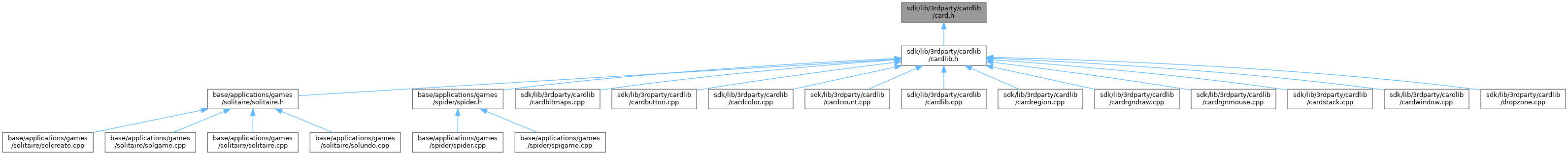ReactOS 0.4.15-dev-6056-gb29b268
card.h File Reference
This graph shows which files directly or indirectly include this file:Go to the source code of this file.

class  Card

## Enumerations

enum  eSuit { Clubs = 0 , Diamonds = 1 , Hearts = 2 , Spades = 3 }

enum  eValue {
Ace = 1 , Two = 2 , Three = 3 , Four = 4 ,
Five = 5 , Six = 6 , Seven = 7 , Eight = 8 ,
Nine = 9 , Ten = 10 , Jack = 11 , Queen = 12 ,
King = 13
}

## Functions

int MAKE_CARD (int Value, int Suit)

## ◆ eSuit

Enumerator
Clubs
Diamonds
Hearts

Definition at line 11 of file card.h.

11{ Clubs = 0, Diamonds = 1, Hearts = 2, Spades = 3 };
@ Clubs
Definition: card.h:11
@ Hearts
Definition: card.h:11
@ Diamonds
Definition: card.h:11
Definition: card.h:11

## ◆ eValue

Enumerator
Ace
Two
Three
Four
Five
Six
Seven
Eight
Nine
Ten
Jack
Queen
King

Definition at line 12 of file card.h.

12 { Ace = 1, Two = 2, Three = 3, Four = 4, Five = 5, Six = 6, Seven = 7,
13 Eight = 8, Nine = 9, Ten = 10, Jack = 11, Queen = 12, King = 13 };
@ Queen
Definition: card.h:13
@ Nine
Definition: card.h:13
@ Three
Definition: card.h:12
@ King
Definition: card.h:13
@ Jack
Definition: card.h:13
@ Ten
Definition: card.h:13
@ Four
Definition: card.h:12
@ Eight
Definition: card.h:13
@ Two
Definition: card.h:12
@ Ace
Definition: card.h:12
@ Six
Definition: card.h:12
@ Seven
Definition: card.h:12
@ Five
Definition: card.h:12

## ◆ MAKE_CARD()

 int MAKE_CARD ( int Value, int Suit )
inline

Definition at line 15 of file card.h.

16{
17 if(Value < 1) Value = 1;
18 if(Value == 14) Value = 1;
19 if(Value > 13) Value = 13;
20
21 if(Suit < 0) Suit = 0;
22 if(Suit > 3) Suit = 3;
23
24 return ((Value - 1) * 4 + Suit);
25}
_Must_inspect_result_ _In_ WDFKEY _In_ PCUNICODE_STRING _Out_opt_ PUSHORT _Inout_opt_ PUNICODE_STRING Value
Definition: wdfregistry.h:413

Referenced by Card::Card().